A propagation of optical wave in an optical fiber is represented by the Maxwell’s equations. Generally, rapidly varying components of electric field are ignored and a behavior of optical pulse is approximated by a slowly varying envelope curve in order to simplify the calculation. In case a time system of group velocity of pulse (decay coordinate system) is utilized, a propagation equation for an envelope function of optical pulse, A(z,T), is expressed as follows.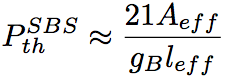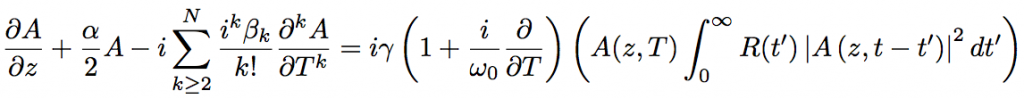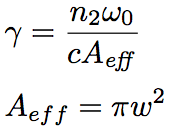γ is a nonlinear coefficient. This equation is called as nonlinear Schroedinger equation and is often used for a description of ultrashort pulse propagation in an optical fiber. In the left side of the equation above, the second term represents a fiber loss, and the third term represents an effect of wavelength dispersion including higher-order dispersions. The right side of the equation above represents nonlinear optical effects such as SPM, FWM, self-steeping, and SRS. R(t) is a Raman response function, and is contributed by both electronic and vibrational (for Raman) transitions. Fig. 1 shows a delayed Raman response function, hR(t), calculated from the Raman gain spectrum in Fig. 1 (an experimental result).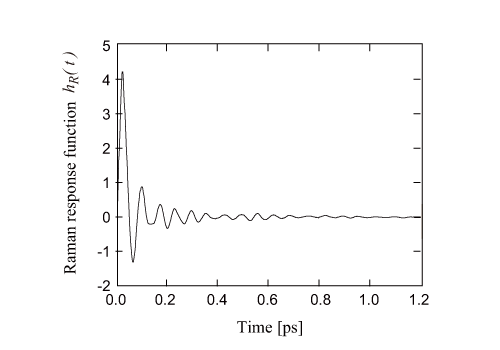Fig. 1 Raman gain spectrum of silica optical fiber

Assuming that an electronic transition occurs instantaneously, a Raman response function, R(t), is expressed by the following equation,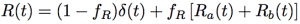where fR is a contribution ratio of delayed Raman response given by Ra(t)+Rb(t). Ra(t) and Rb(t) are Raman response functions corresponding to ga and gb shown in Fig. 2, respectively. ga and gb are a couple of decomposed components of parallel Raman gain, g||. g is a perpendicular Raman gain.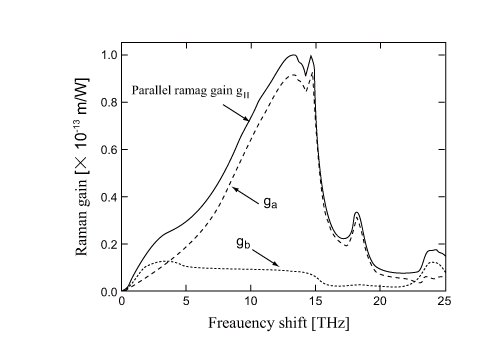Fig 2. Parallel Raman gain spectrum

The Raman response function Ra(t) can be approximated by the following expressions,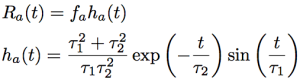where τ1 and τ2 are regulation parameters. τ1 = 12.2 fs, τ2 = 32 fs, and fs = 0.75 are adopted since they are very close to a practical Raman gain spectrum. The Raman response function Rb(t) can be approximated by the following expressions.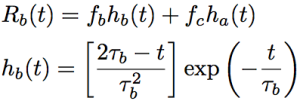Provided fb = 0.21, fc = 0.04, and τb = 96 fs, and fR = 0.245, the Raman response function R(t) is properly approximated in the frequency range of 0~15 THz.

Thus, the nonlinear Schroedinger equation includes higher-order dispersions and nonlinear optical effects, and accurately reproduces an effect of delayed Raman response. Therefore, by using the nonlinear Schroedinger equation , it is possible to accurately analyze a temporal evolution of ultrashort pulse with a duration of 20~30 fs. However, for a pulse shorter than10 fs, since the slowly varying envelope approximation is broken down, the nonlinear Schroedinger equation  is not usable. For this ultrashort pulse, the Maxwell’s equations must be directly solved by using numerical integral.

For a pulse with a duration with ca. 50 fs or shorter, the nonlinear Schroedinger equation can be simplified as below.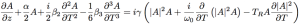By applying the Taylor expansion to |A(z,t-t’)|2 in the nonlinear Schroedinger equation and considering the zeroth- and first-order terms of t’, the following relationship is obtained.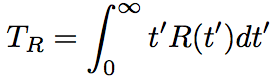TR is associated with a slope of Raman gain spectrum, and is mostly ~5 fs. The first, second, and third terms of right side of the nonlinear Schroedinger equation represent self phase modulation, self steeping, and Raman effect, respectively.